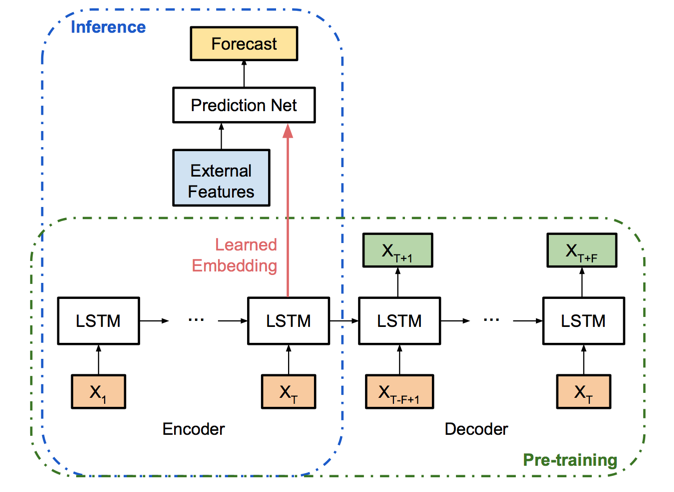# Model converges on training but output at test time is constant

Hi!

I’m trying to implement the model presented on this paper by Uber: https://arxiv.org/pdf/1709.01907.pdf.

Long story short, I have a LSTM used to predict the next day of a time series, after consuming N days of data.I have a function used to train that inference part, and another one to infer the next value on the series on test time. They are identical, but the second one doesn’t backpropagate or train the model. When I’m training the inference model the model quickly converges and I can extract it’s outputs at train time, they are something like that:

(removed image as new users can only post one image)

But when I run the model on test time, the output remains the same! And exactly equal to last output of the training phase!

I’ve tried everything on the last days, even rewrote the entire inference function to no success. I finally discovered that just by activating the optimizer step again the output starts to change. But the moment the test is done without optimization and grads the output freezes no matter the input, even with random vectors as input!

I’m really desperate, I would be very grateful even for some possible direction to tackle this problem from. Here is the code of the training function and the forecasting function (test time). Both of them use the inference part of the model!

FORECASTING FUNCTION (always same output)

``````
def ForecastSequence1x12(encoder, forecaster, window_size, dev_pairs,num_stochastic):

# number of stochastic predictions MC dropout
B = num_stochastic
#encoder.eval()
total_loss = 0
outputs = []
real_values = []
hiddens = []
for iter in range(1,len(dev_pairs)):
list_predictions = []
input_tensor = dev_pairs[iter - 1]
target_tensor = dev_pairs[iter - 1]

encoder_hidden1 = encoder.initHidden()
_,(ht,ct) = encoder(
target_tensor[:window_size], encoder_hidden1, use_dropout=False)

hidden_and_input = torch.cat((ht.squeeze(),
ct.squeeze(),
input_tensor[window_size]
))

forecaster_output = forecaster(hidden_and_input ,use_dropout=False)

outputs += [forecaster_output.cpu().numpy()]
real_values += [target_tensor[window_size].cpu().numpy().squeeze()]
total_loss += (forecaster_output.cpu().numpy() - target_tensor[window_size].cpu().numpy().squeeze())**2

print(total_loss/len(dev_pairs))

return outputs,real_values
``````

TRAINING FUNCTION

``````def TrainForecast(input_tensor, target_tensor, encoder, forecaster,
encoder_optimizer, forecaster_optimizer, criterion,
window_size):

input_length = input_tensor.size(0)
target_length = target_tensor.size(0)

loss = 0
#print(torch.mean(target_tensor[:window_size]))
encoder_hidden = encoder.initHidden()
_,encoder_hidden = encoder(
target_tensor[:window_size], encoder_hidden, use_dropout=False)
# concatenate hidden state and input_tensor (exogenous variables to the time series)
hidden_and_input = torch.cat((encoder_hidden.squeeze(),
encoder_hidden.squeeze(),
input_tensor[window_size]))
#print(torch.mean(hidden_and_input))
#print("forecaster_input",hidden_and_input)
forecaster_output = forecaster(hidden_and_input,use_dropout=False)
#after all timesteps have been processed by the encoder, we check error only with last real target
loss = criterion(forecaster_output.squeeze(), target_tensor[window_size].squeeze())
#print(forecaster_output,target_tensor[days_window])
loss.backward()

encoder_optimizer.step()
forecaster_optimizer.step()

return (loss.item() / target_length), forecaster_output.detach().cpu().numpy().squeeze()
``````

I just skimmed through your code, and stumbled over these lines:

``````list_predictions += [forecaster_output.cpu().numpy() ]#+ target_tensor.numpy()]
# pass list of lists with lists of B predictions
outputs += [list_predictions]
``````

Wouldn’t this just add the first prediction to `outputs`, while the new ones are appended at the end?
This wouldn’t explain why your output changes, when the optimizer is called, so I probably miss something.

Yeah, you are right. When the model is functional I hope to use Monte Carlos Dropout so I would need multiple computations of the same prediction. But for now I’m just appending the first prediction to test. I will clean up the code on my postI’m not sure, if that was the issue or not.Do you get different predictions now?

Same thing… This wasn’t the problem. I’ve updated the code on my post without that part to make it less confusing.

Did you try to use the same data from training while testing, as a sanity check?

Yes! This is all done with the training data. I haven’t touched the dev data yet